Courses

# MCQ (Previous Year Questions) - States Of Matter (Level 2)

## 10 Questions MCQ Test Chemistry for JEE Advanced | MCQ (Previous Year Questions) - States Of Matter (Level 2)

Description
This mock test of MCQ (Previous Year Questions) - States Of Matter (Level 2) for JEE helps you for every JEE entrance exam. This contains 10 Multiple Choice Questions for JEE MCQ (Previous Year Questions) - States Of Matter (Level 2) (mcq) to study with solutions a complete question bank. The solved questions answers in this MCQ (Previous Year Questions) - States Of Matter (Level 2) quiz give you a good mix of easy questions and tough questions. JEE students definitely take this MCQ (Previous Year Questions) - States Of Matter (Level 2) exercise for a better result in the exam. You can find other MCQ (Previous Year Questions) - States Of Matter (Level 2) extra questions, long questions & short questions for JEE on EduRev as well by searching above.
QUESTION: 1

Solution:
QUESTION: 2

Solution:
QUESTION: 3

### The ratio of the rate of diffusion of helium and methane under identical condition of pressure and temperature will be          [JEE 2005]

Solution:
QUESTION: 4

The given graph represents the variation of Z (compressibility factor =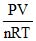) versus P, for three real gases A, B and C. Identify the only incorrect statement.                  [JEE 2006]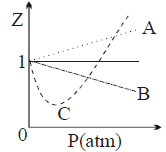Solution:
QUESTION: 5

Match gases under specific conditions listed in Column I with their properties / laws in Column II. Indicate your answer by darkening the appropriate bubbles of the 4 × 4 matrix given in the ORS.                     [JEE 2007]

Column I

(A) Hydrogen gas (P = 200 atm, T = 273 K)

(B) Hydrogen gas (P ~ 0, T = 273 K)

(C) CO2 (P = 1 atm, T = 273 K)

(D) Real gas with very large molar volume

Column II

(P) Compressibility factor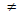1

(Q) Attractive forces are dominant

(R) PV = nRT

(S) P (V - nb) = nRT

Solution:
*Multiple options can be correct
QUESTION: 6

A gas described by van der Waals equation             [JEE 2008]

Solution: (A, C, D)At low pressure, when the sample occupies a large volume, the molecules are so far apart for most of the time that the intermolecular forces play no significant role, and the gas behaves virtually perfectly.
*Multiple options can be correct
QUESTION: 7

For one mole of a van der Waals gas when b = 0 and T = 300 K, the PV vs. l/V plot is shown below. The value of the van der Waals constant a (atm. liter2 mol-2) is                           [JEE 2012]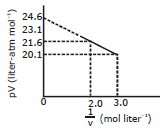Solution:
QUESTION: 8

A fixed mass `m' of a gas is subjected to transformation of states from K to L to M to N and back to K as shown in the figure.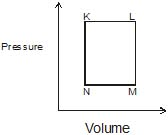The succeeding operations that enable this transformation of states are           [JEE (ADVANCED)-2013,3/120]

Solution:
QUESTION: 9

A fixed mass `m' of a gas is subjected to transformation of states from K to L to M to N and back to K as shown in the figure.The pair of isochoric processes among the transformation of states is :            [JEE (ADVANCED)-2013,3/120]

Solution:
QUESTION: 10

A fixed mass `m' of a gas is subjected to transformation of states from K to L to M to N and back to K as shown in the figure.Two non-reactive monoatomic ideal gases have their atomic masses in the ratio 2 : 3. The ratio of their partial pressures, when enclosed in a vessel kept at a constant temperature, is 4 : 3. The ratio of their densities is